## [ITOC-ch6] ADVANCED TOPICS IN COMPUTABILITY THEORY

### 6.1 THE RECURSION THEOREM

Making machines that reproduce themselves.

SELF-REFERENCE

Construct $SELF$ prints out a copy of its own description.

LEMMA 6.1

define computable function $q : Σ^ ∗\longrightarrow Σ ^ ∗$

• w is any string, $q(w) = < P_w >$
• $P_w$ is a TM that prints out w and then halts.

$SELF$

• 2 parts: $A$ and $B$, so $< SELF > = < AB >$
•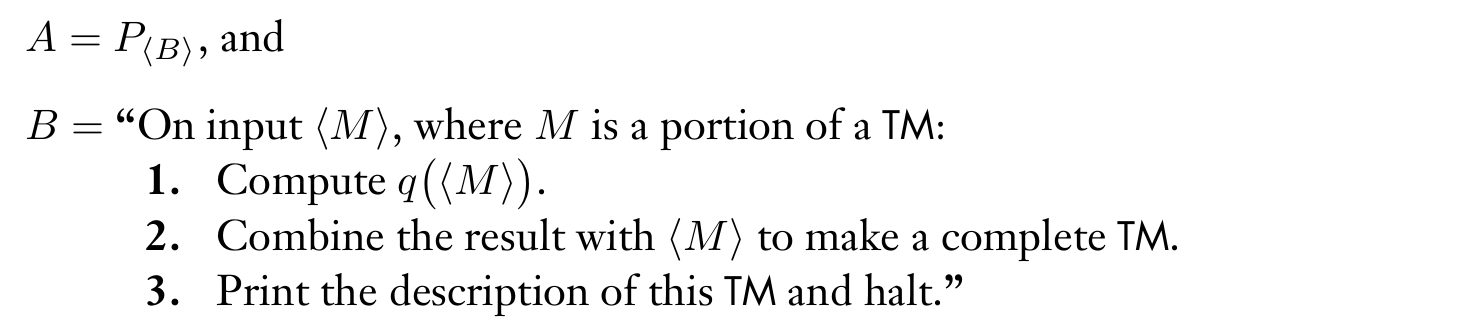RZ师兄的例子

Extends the technique so that a program can automatically obtain its own description and then go on to compute with it

Recursion theorem

• First we have a T, than we can construct R
• Input w to R, R can obtain < R > and give < R > and w to T for further computation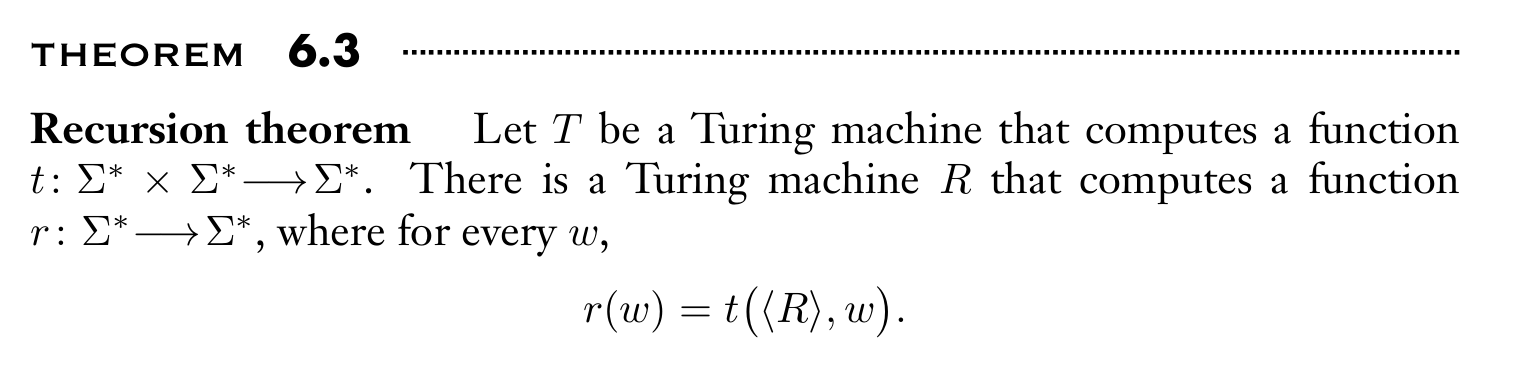$A$ writes $w < BT >$ , $B$ writes $< A >$ , together the resulting string $< R, w >$ is on the tape, than passes control to T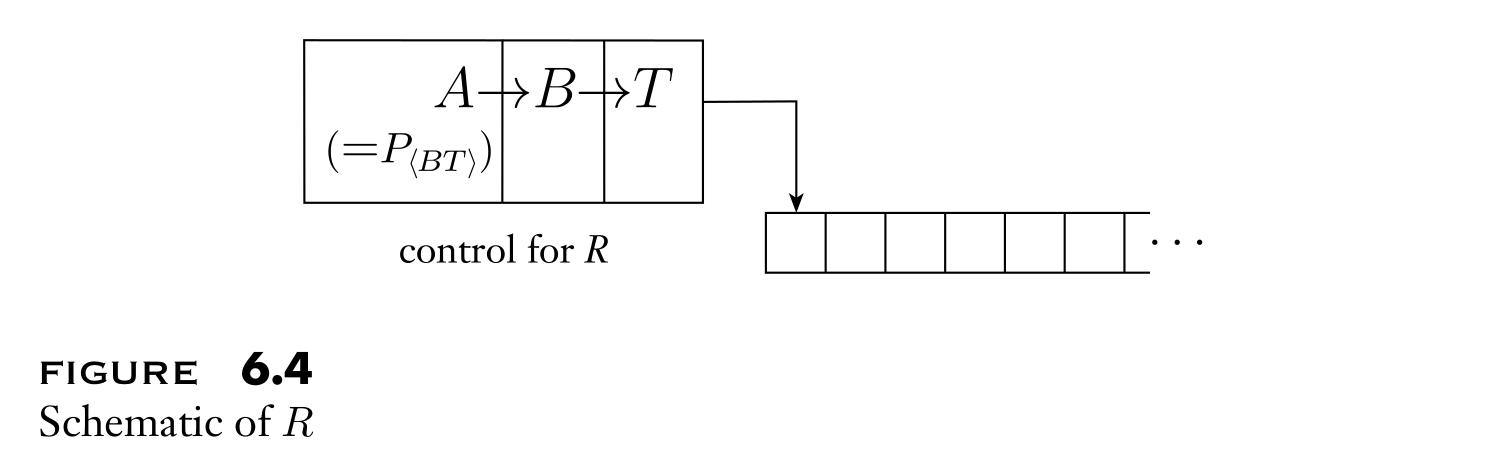APPLICATIONS

• computer virus
• Simpler proof of $A_{TM}$ is undecidable (4.11显式构建了D出来)
• $MIN_{TM}$ is not Turing-recognizable.
• For computable function $t: Σ ^ ∗ → Σ^ ∗$, there is a $< F > = t( < F > )$

### 6.2 DECIDABILITY OF LOGICAL THEORIES

model

• a tuple $(U, P_1 , . . . , P_k )$, where U is the universe ( possible variable values ) and $P_1$ through $P_k$ are the relations assigned to symbols $R_1$ through $R_k$.

language of a model

• use things only the model defined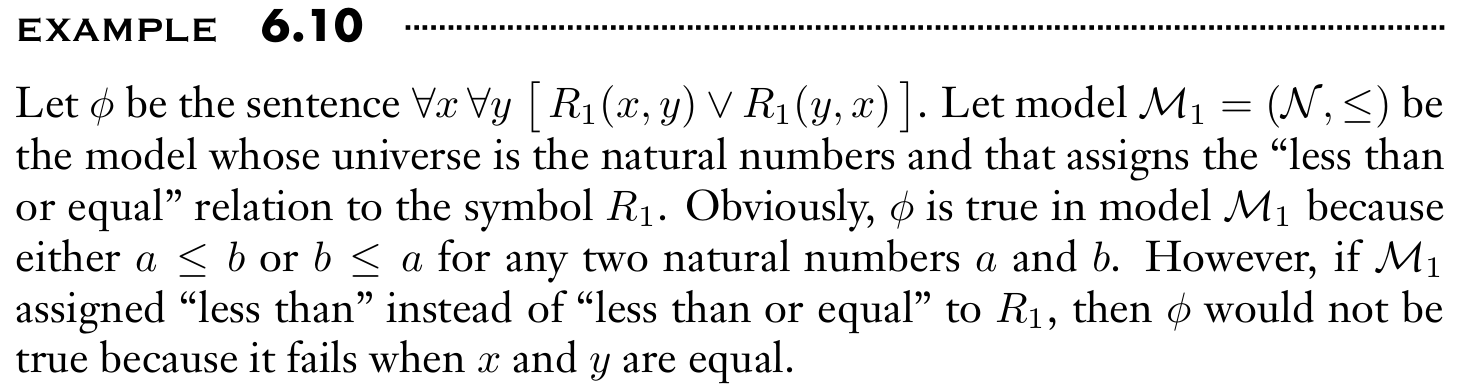theory of M $Th(M)$

• the collection of true sentences in the language of that model

6.12 $Th(N , +)$ is decidable.

6.13 $Th(N , +, ×)$ is undecidable.

6.16 Some true statement in $Th(N , +, ×)$ is not provable.

(真假性客观存在但不可证明)

### 6.3 TURING REDUCIBILITY

Reducibility

• if A is reducible to B, and we find a solution to B, we can obtain a solution to A.

Mapping reducibility

• $\exists f$ so $w \in A \Longleftrightarrow f(w) \in B$

Turing reducibility

oracle for a language

• an external device that can report whether a string w is a member of the language

oracle Turing Machine

• TM that has the additional capability of querying an oracle.
• more powerful than TM
• Still cannot decide all languages

$E_{TM}$ is decidable relative to $A_{TM}$

• we can use the oracle of $A_{TM}$ to decide $E_{TM}$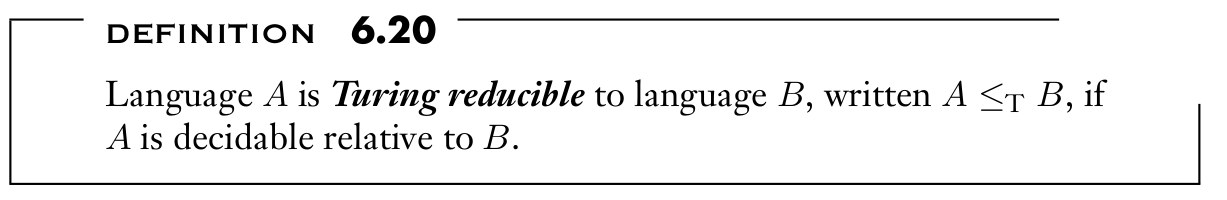### 6.4 A DEFINITION OF INFORMATION

define information using computability theory.

quantity of information

• the size of that object’s smallest representation or description.

MINIMAL LENGTH DESCRIPTIONS

Select our language describing information

• describe a binary string x as $< M > w$ while M is a TM and w is input
• locate the separation between $< M >$ and $w$
• One way to do so is to write each bit of $< M >$ twice, writing 0 as 00 and 1 as 11, and then follow it with 01 to mark the separation point.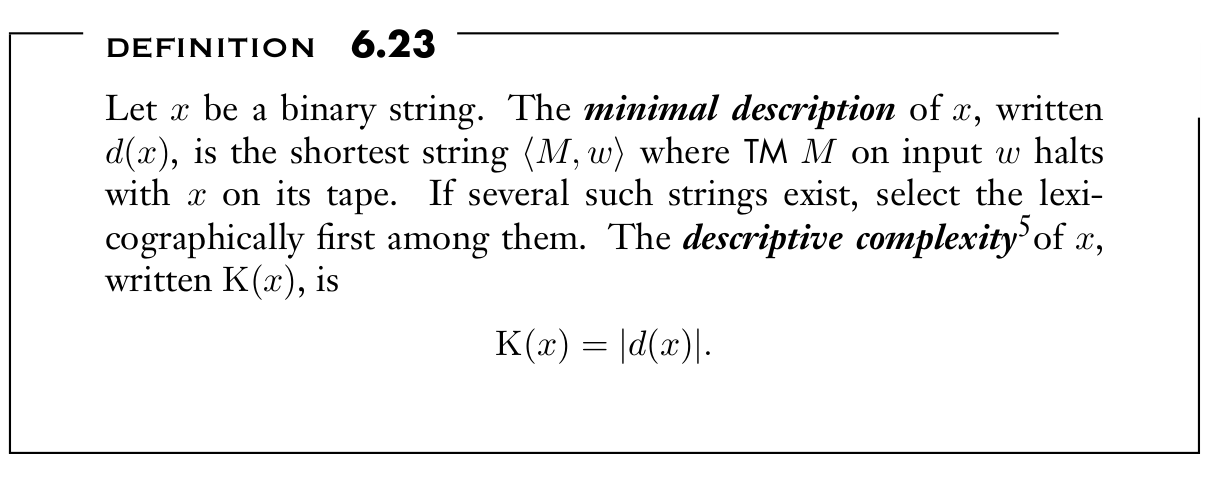6.24: $\exists c \forall x \ [ K(x) \le |x| + c ]$

6.25: $\exists c \forall x \ [ K(xx) \le K(x) + c ]$

6.26: $\exists c \forall x,y \ [ K(xy) \le 2K(x) + K(y) + c ]$